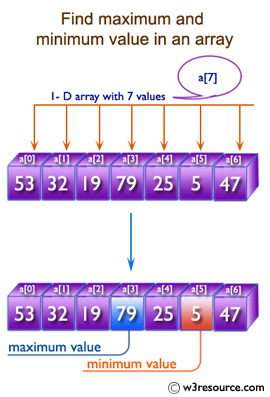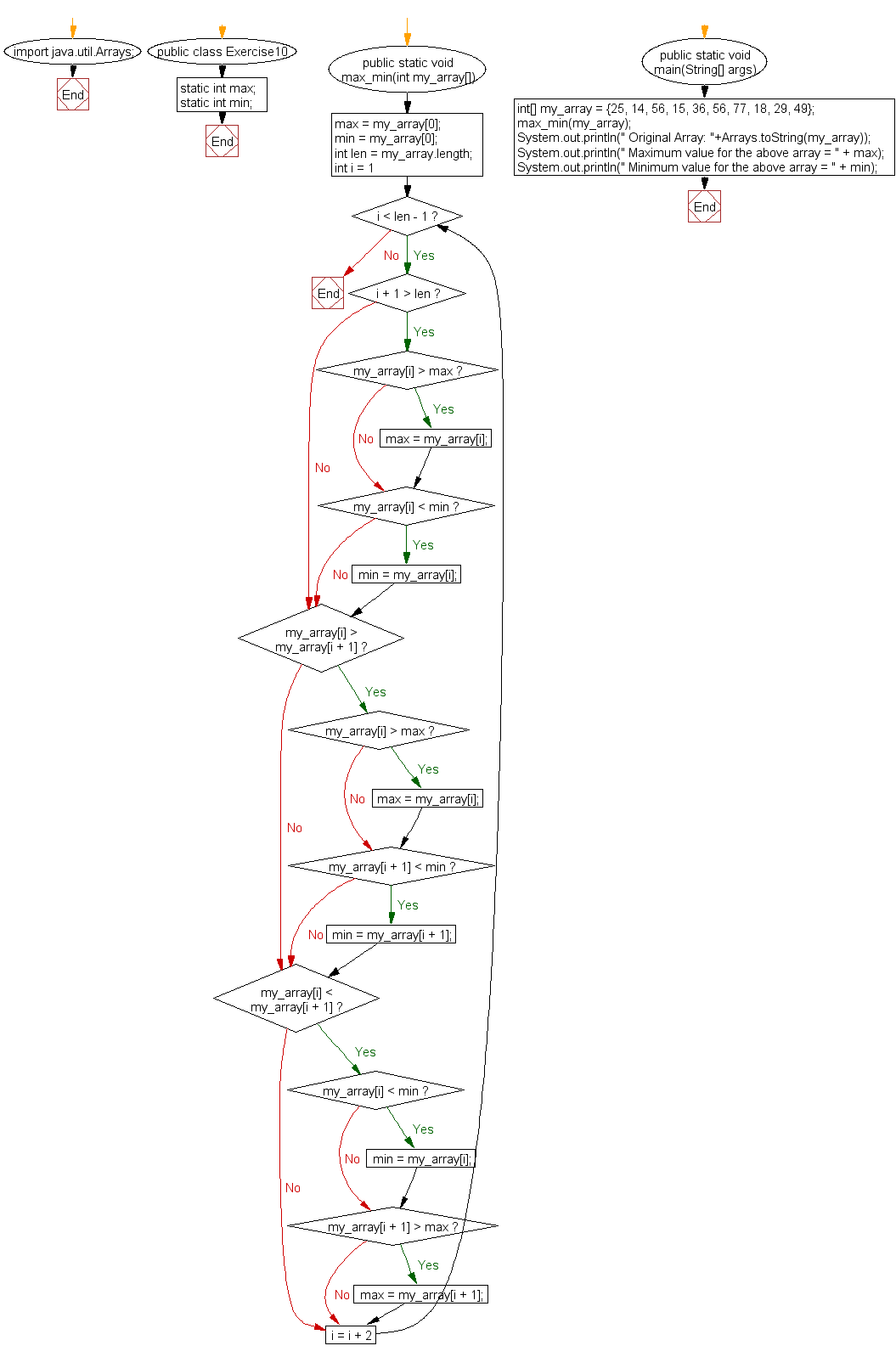﻿ Java exercises: Find the maximum and minimum value of an array - w3resource# Java Array Exercises: Find the maximum and minimum value of an array

## Java Array: Exercise-10 with Solution

Write a Java program to find the maximum and minimum value of an array.

Pictorial Presentation:Sample Solution:

Java Code:

``````import java.util.Arrays;
public class Exercise10 {

static int max;
static int min;

public static void max_min(int my_array[]) {
max = my_array;
min = my_array;
int len = my_array.length;
for (int i = 1; i < len - 1; i = i + 2) {
if (i + 1 > len) {
if (my_array[i] > max) max = my_array[i];
if (my_array[i] < min) min = my_array[i];
}
if (my_array[i] > my_array[i + 1]) {
if (my_array[i] > max) max = my_array[i];
if (my_array[i + 1] < min) min = my_array[i + 1];
}
if (my_array[i] < my_array[i + 1]) {
if (my_array[i] < min) min = my_array[i];
if (my_array[i + 1] > max) max = my_array[i + 1];
}
}
}

public static void main(String[] args) {
int[] my_array = {25, 14, 56, 15, 36, 56, 77, 18, 29, 49};
max_min(my_array);
System.out.println(" Original Array: "+Arrays.toString(my_array));
System.out.println(" Maximum value for the above array = " + max);
System.out.println(" Minimum value for the above array = " + min);
}
}
```
```

Sample Output:

```Original Array: [25, 14, 56, 15, 36, 56, 77, 18, 29, 49]
Maximum value for the above array = 77
Minimum value for the above array = 14
```

Flowchart:Visualize Java code execution (Python Tutor):

Java Code Editor:

Improve this sample solution and post your code through Disqus

What is the difficulty level of this exercise?

Test your Programming skills with w3resource's quiz.

﻿

## Java: Tips of the Day

countOccurrences

Counts the occurrences of a value in an array.

Use Arrays.stream().filter().count() to count total number of values that equals the specified value.

```public static long countOccurrences(int[] numbers, int value) {
return Arrays.stream(numbers)
.filter(number -> number == value)
.count();
}
```

Ref: https://bit.ly/3kCAgLb